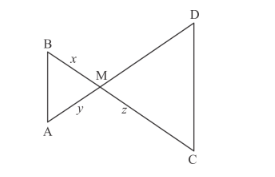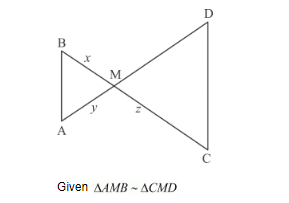# In the given figure, ∆AMB ∼ ∆CMD; determine MD in terms of x, y and z.

Question:

In the given figure, ∆AMB ∼ ∆CMD; determine MD in terms of xy and z.Solution:

We are given ∆AMB ∼ ∆CMD

We have to determine the value of MD in terms of x, y and z.$\Rightarrow \frac{B M}{M D}=\frac{A M}{C M}$

$\frac{x}{M D}=\frac{y}{z}$

By cross multiplication we get $M D=\frac{x z}{y}$

Hence, the value of $M D$ is $\frac{x z}{y}$.